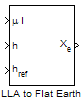# LLA to Flat Earth

Estimate flat Earth position from geodetic latitude, longitude, and altitude

• Library:
• Aerospace Blockset / Utilities / Axes Transformations

•## Description

The LLA to Flat Earth block converts a geodetic latitude $\left(\overline{\mu }\right)$, longitude $\left(\overline{\iota }\right)$, and altitude (h) into a 3-by-1 vector of flat Earth position $\left(\overline{p}\right)$. Latitude and longitude values can be any value. However, latitude values of +90 and -90 may return unexpected values because of singularity at the poles. For more information on the flat Earth coordinate system, see Algorithms.

## Limitations

• This estimation method assumes that the flight path and bank angle are zero.

• This estimation method assumes the flat Earth z-axis is normal to the Earth at the initial geodetic latitude and longitude only. This method has higher accuracy over small distances from the initial geodetic latitude and longitude, and nearer to the equator. The longitude has higher accuracy with smaller variations in latitude. Additionally, longitude is singular at the poles.

## Ports

### Input

expand all

Geodetic latitude and longitude, specified as a 2-by-1 vector, in degrees.

Data Types: `double`

Altitude above the input reference altitude, specified as a scalar, in the same units as the flat Earth position.

Data Types: `double`

Reference height from the surface of the Earth to the flat Earth frame, specified as a scalar, in the same units as the flat Earth position. The reference height is estimated with regard to Earth frame.

Data Types: `double`

Reference location, specified as a 2-by-1 vector, in degrees of latitude and longitude, for the origin of the estimation and the origin of the flat Earth coordinate system. Use this port if you want to specify the reference location as a dynamic value.

#### Dependencies

To enable this port, select Input reference position and orientation.

Data Types: `double`

Angle, specified as a scalar, for converting flat Earth x and y coordinates to North and East coordinates. Use this port if you want to specify the angle as a dynamic value.

#### Dependencies

To enable this port, select Input reference position and orientation.

Data Types: `double`

### Output

expand all

Position in flat Earth frame, returned as a vector.

Data Types: `double`

## Parameters

expand all

Parameter and output units:

Units

Position

Altitude

`Metric (MKS)`

Meters

Meters

Meters

`English`

Feet

Feet

Feet

#### Programmatic Use

 Block Parameter: `units` Type: character vector Values: ```'Metric (MKS)'``` | `'English'` Default: ```'Metric (MKS)'```

Planet model to use, `Custom` or `Earth (WGS84)`.

#### Dependencies

Selecting the `Custom` option disables the Units parameter and enables these parameters:

• Flattening

#### Programmatic Use

 Block Parameter: `ptype` Type: character vector Values: ```'Earth (WGS84)'``` | `'Custom'` Default: ```'Earth (WGS84)'```

Flattening of the planet, specified as a double scalar.

#### Dependencies

To enable this parameter, set Planet model to `Custom`.

#### Programmatic Use

 Block Parameter: `F` Type: character vector Values: double scalar Default: `1/298.257223563`

Radius of the planet at its equator, in the same units as the desired units for ECEF position.

#### Dependencies

To enable this parameter, set Planet model to `Custom`.

#### Programmatic Use

 Block Parameter: `R` Type: character vector Values: double scalar Default: `6378137`
• To enable input ports for reference position and angle to convert flat Earth, select this check box.

• To specify the reference positions and angle as static values, clear this check box.

Select this check box if you want

#### Programmatic Use

 Block Parameter: `refPosPort` Type: character vector Values: `'off'` | `'on'` Default: `'off'`

Reference location in latitude and longitude, specified as 2-by-1 vector, in degrees.

#### Dependencies

To enable this parameter, clear Input reference position and orientation.

#### Programmatic Use

 Block Parameter: `LL0` Type: character vector Values: 2-by-1 vector Default: `[0 10]`

Angle to convert flat Earth x and y coordinates to North and East coordinates, specified as a scalar double, in degrees.

#### Dependencies

To enable this parameter, clear Input reference position and orientation.

#### Programmatic Use

 Block Parameter: `psi` Type: character vector Values: double scalar Default: `0`

## Algorithms

The flat Earth coordinate system assumes the z-axis is downward positive. The estimation begins by finding the small changes in latitude and longitude from the output latitude and longitude minus the initial latitude and longitude.

`$\begin{array}{l}d\mu =\mu -{\mu }_{0}\\ d\iota =\iota -{\iota }_{0}\end{array}$`

To convert geodetic latitude and longitude to the North and East coordinates, the estimation uses the radius of curvature in the prime vertical (RN) and the radius of curvature in the meridian (RM). RN and RM are defined by the following relationships:

`$\begin{array}{l}{R}_{N}=\frac{R}{\sqrt{1-\left(2f-{f}^{2}\right){\mathrm{sin}}^{2}{\mu }_{0}}}\\ {R}_{M}={R}_{N}\frac{1-\left(2f-{f}^{2}\right)}{1-\left(2f-{f}^{2}\right){\mathrm{sin}}^{2}{\mu }_{0}}\end{array}$`

where (R) is the equatorial radius of the planet and $f$ is the flattening of the planet.

Small changes in the North (dN) and East (dE) positions are approximated from small changes in the North and East positions by

`$\begin{array}{l}dN=\frac{d\mu }{\text{atan}\left(\frac{1}{{R}_{M}}\right)}\\ dE=\frac{d\iota }{\text{atan}\left(\frac{1}{{R}_{N}\mathrm{cos}{\mu }_{0}}\right)}\end{array}$`

With the conversion of the North and East coordinates to the flat Earth x and y coordinates, the transformation has the form of

`$\left[\begin{array}{c}{p}_{x}\\ {p}_{y}\end{array}\right]=\left[\begin{array}{cc}\mathrm{cos}\psi & \mathrm{sin}\psi \\ -\mathrm{sin}\psi & \mathrm{cos}\psi \end{array}\right]\left[\begin{array}{c}N\\ E\end{array}\right],$`

where

`$\left(\psi \right)$`

is the angle in degrees clockwise between the x-axis and north.

The flat Earth z-axis value is the negative altitude minus the reference height (href):

`${p}_{z}=-h-{h}_{ref}.$`

 Stevens, B. L., and F. L. Lewis. Aircraft Control and Simulation, Hoboken, NJ: John Wiley & Sons, 2003.

 Etkin, B. Dynamics of Atmospheric Flight Hoboken, NJ: John Wiley & Sons, 1972.

## Support평가판 신청